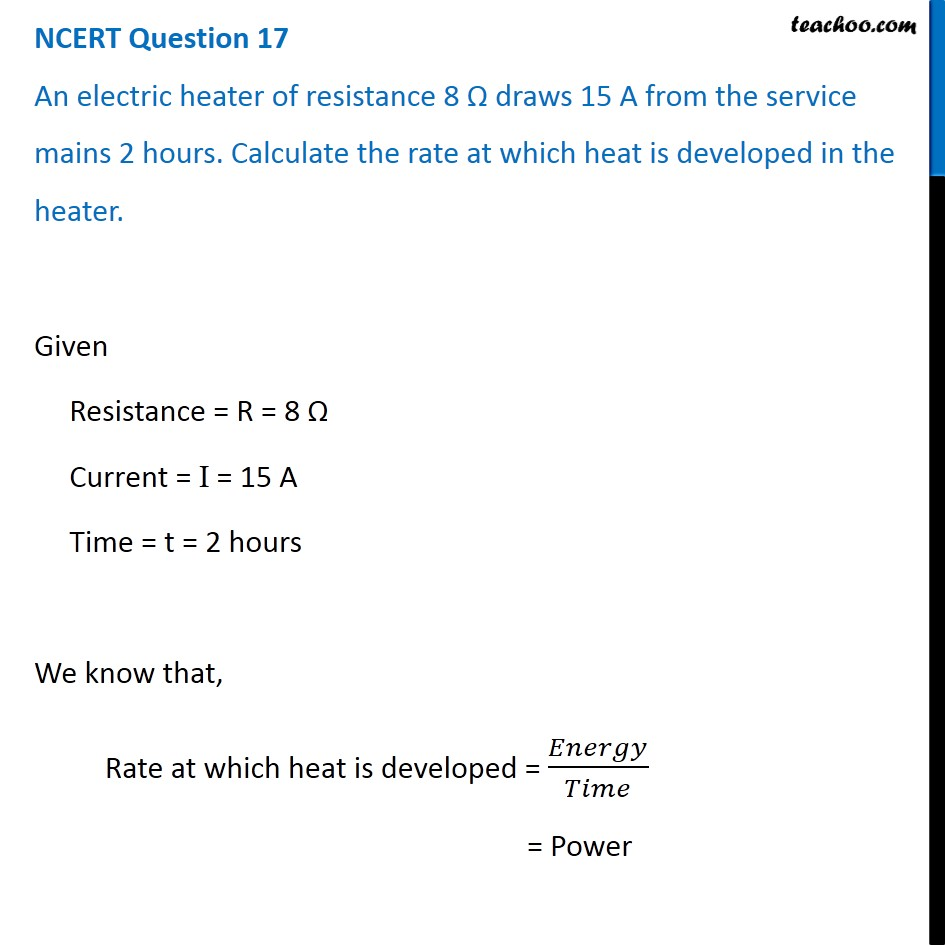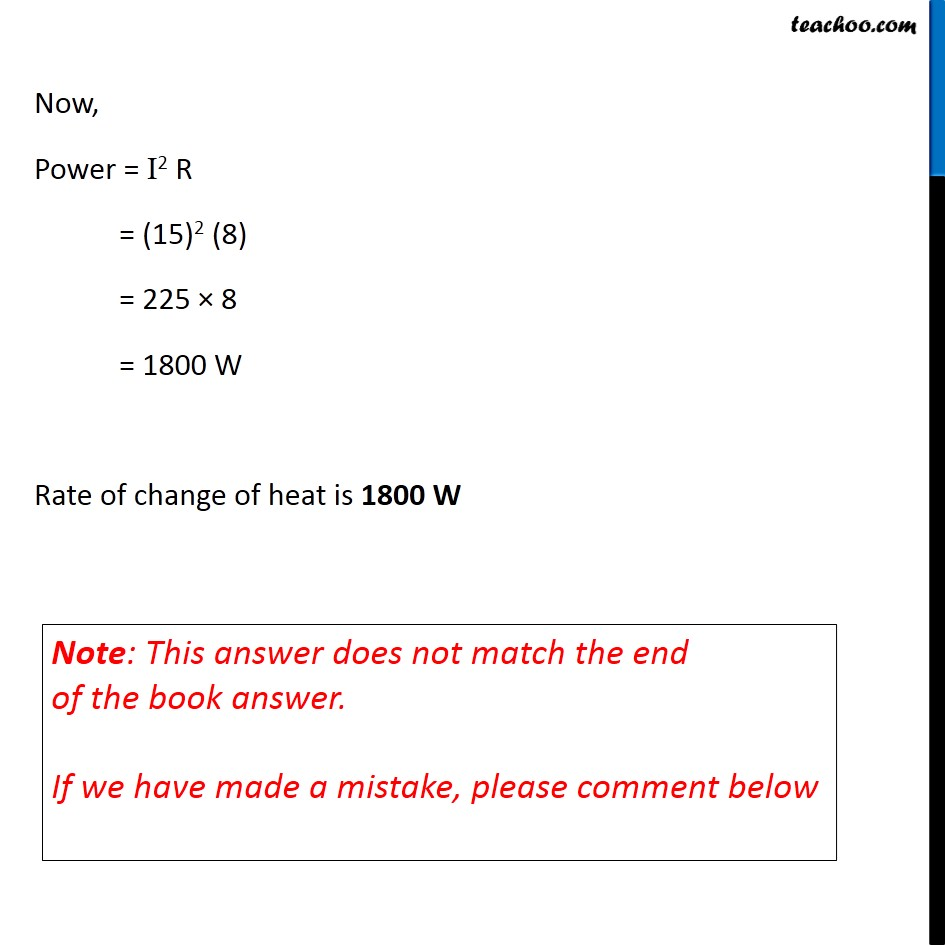NCERT Questions

Class 10
Chapter 12 Class 10 - Electricity

## An electric heater of resistance 8 Ω draws 15 A from the service mains 2 hours. Calculate the rate at which heat is developed in the heater.Learn in your speed, with individual attention - Teachoo Maths 1-on-1 Class

### Transcript

NCERT Question 17 An electric heater of resistance 8 Ω draws 15 A from the service mains 2 hours. Calculate the rate at which heat is developed in the heater. Given Resistance = R = 8 Ω Current = I = 15 A Time = t = 2 hours We know that, Rate at which heat is developed = 𝐸𝑛𝑒𝑟𝑔𝑦/𝑇𝑖𝑚𝑒 = Power Now, Power = I2 R = (15)2 (8) = 225 × 8 = 1800 W Rate of change of heat is 1800 W Note: This answer does not match the end of the book answer. If we have made a mistake, please comment below